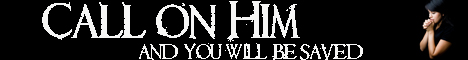Note:  Do not rely on this information. It is very old.

# Rotor

Rotor. If a rigid body undergo a process of translation only, all points in it move through equal distances along lines which are parallel, i.e. in the same direction. Hence the motion may be represented by a straight line of definite length and direction drawn anywhere - i.e. by a vector. The direction of an axis of rotation and the angular velocity are not, however, sufficient to determine the motion of a rotating body, for the position of the axis will affect the result. Obviously equal rotations about i parallel axes are not the same thing. Hence, to represent a rotation, we must have a line of definite length, direction, and position: This is therefore a localised vector, and was termed by Clifford a rotor, the word being given to it in consequence of its connection with the theory of rotating bodies. A force acting on a solid body is a rotor, for it will lead to a different result whether the force act along one particular line or along a parallel to it, the difference between the two results being expressed as a definite couple. A vector remains unaltered wherever it may move in space, provided it keep parallel to itself, but two rotors are only identical when they are in the .same line and of the same length and sense. The addition of rotors is affected in the same way as the composition of forces or of rotations, and just as the com. position of a number of rotations about any axes gives usually not a simple rotation, but a perfectly general motion of the body, so the result of rotor addition is generally not a simple rotor.